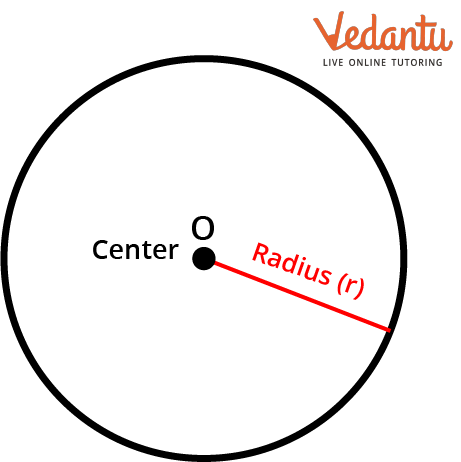Courses
Courses for Kids
Free study material
Offline Centres
More

# Applications of a CircleLast updated date: 29th Nov 2023
Total views: 99.6k
Views today: 0.99k## Introduction

Kids, the circle is such a common shape that we encounter it practically everywhere we go, from packing a circle lunch box for the trip to school in the morning to eating circle-shaped cookies when you get home. Adults who look at a watch or bottle cap also notice this shape. Let's learn more about this interesting shape and its applications.

## What is a Circle?

The term "circle" is derived from the Greek word "kirkos," meaning hoop or ring. A circle can be defined as a two-dimensional figure whose boundary consists of points equidistant from the centre of the circle. Many people believe that the circle is perfect. The circle is ideal because every line crossing its centre produces a reflection.Circle

## Applications of Circle

The fixed distance from the centre is called the circle's radius. The diameter of the circle is double its radius. The radius of a circle can be used to calculate the perimeter and area of a circle. We have seen many shapes that are round in shape in real life. The use of a circle starts from the pen's tip and ends with the planet's shape. Camera lenses, Ferris wheels, pizza, rings, steering wheels, pies, cakes, and buttons are examples of real-life circle applications.

### Following are the Applications of the Area of a Circle in Daily Life

• To find any cylinder's volume and surface area.

• To find the surface area and volume of a cone.

• To find the surface area and volume of a sphere.

• For taking measurements of various circular figures.

### Application of the Properties of Circle

The properties of a circle are:

1. If the radii of the circles are the same, the circles are said to be congruent.

2. The longest chord in a circle is its diameter.

3. Equal chords subtend equal angles at the centre of the circle.

4. The chord is always divided in half when the radius is drawn perpendicular to it.

5. Circles with different radii are never identical in size but are always similar.

6. Equal in length are the chords equally spaced from the centre.

7. The tangents are parallel if drawn at the diameter's end.

## Questions Related To Circle

1. To find the length of the boundary of a cricket field what can be used?

Ans. The perimeter that can be found using the radius of the circular field.

2. To find the amount of material to be used for the carpeting of a circular room what can be used?

Ans. The area of the circular floor will be useful to estimate the amount of material that will be used for carpeting.

### Conclusion

In this article, you have learnt the definition of circle and the origin of the word “circle”. You have learnt about the application of the circle and its uniqueness. You have also learnt various terms related to circle. You have also understood the application of circle theorems in real life. We hope you liked this article.

## FAQs on Applications of a Circle

1. How to construct a circle?

The construction of a circle step by step -

Instruments required:  A pencil, a ruler, a compass, and paper.

Step 1: Take paper and a compass. Now, placing the compass tip on the paper, Mark a point O.

Step 2:  Take a ruler from mark O, draw a straight line 5 cm from the initial point and mark it A.

Step 3:  Now we can perfectly draw our circle. Aiming from point O to point A, prepare the compass.

Step 4:  From the centre O, draw a perfect 360° angle with the help of a compass.

Our circle is now complete.

2. How do circles form?

A circle is a closed shape that can be created by following a moving point in a plane while keeping a constant distance from one constant point. The Greek word "kirks," which means "hoop" or "ring," is where the word "circle" gets its name.

3. What is the name of a circle with height?

A sagitta is a circle's arc height. It is a line segment with ends at the chord's midway and the arc's midpoint, respectively.